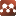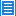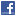## Number Theory## Algorithms for Testing Prime Factors Against Positive Composite Numbers (Finding the Unique Factorization Domain for Said Composite Numbers) in Base 10: a First Course Into Formal Mathematics

Authors: Labib Zakaria

There exist many algorithms to test the primality of positive natural numbers both proved and unproved, as well as in base 10 and outside base 10. Once the primality of a number has been determined, natural questions are \$(1)\$ what the unique prime factors of it are and \$(2)\$ their degree, according to the fundamental theorem of arithmetic. These questions can prove to be useful in beginning to analyze the properties of the number by allowing us to determine the number of (proper) divisors of a number as well as their sum and product. In regards to \$(1)\$, there are many algorithms that could be applied to determine these prime factors through modular arithmetic algorithms. We will be tackling this question in base 10 specifically by constructing functions as curious mathematicians.

Comments: 12 Pages. Hopefully this is obvious from the abstract & a quick overview of the paper, but this is not meant to be an immensely technical paper. It is simply meant to be so that people can nurture an appreciation for math. Constructive criticism appreciated.